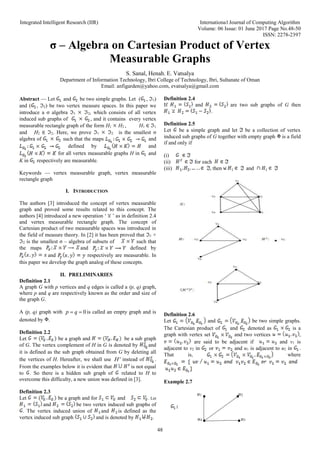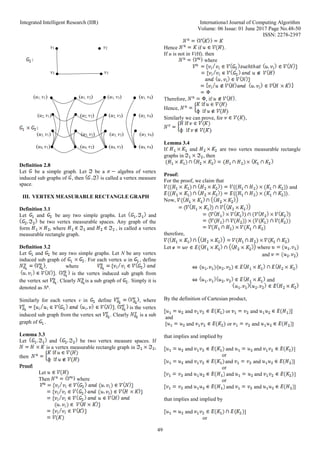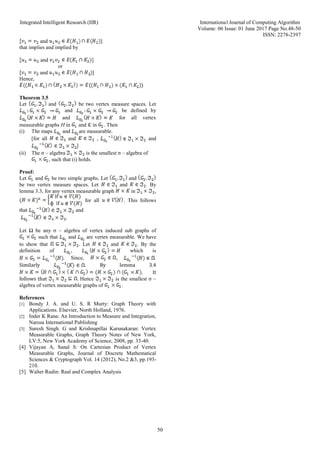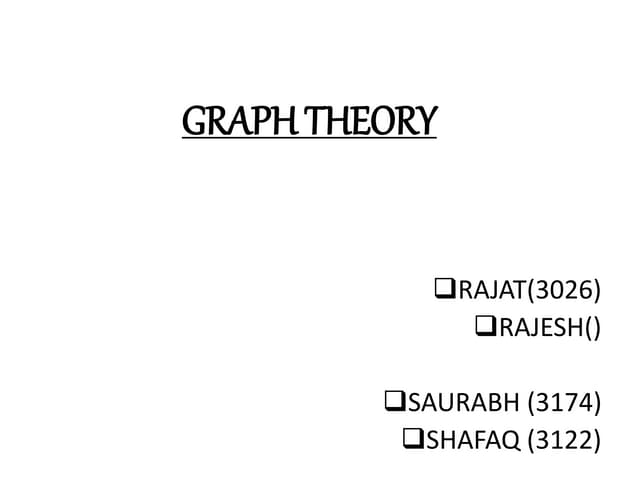PublicitéProchain SlideShareGraph Theory,Graph Terminologies,Planar Graph & Graph Colouring
Chargement dans ... 3
1 sur 3
Publicité

### σ – Algebra on Cartesian Product of Vertex Measurable Graphs

1. Integrated Intelligent Research (IIR) International Journal of Computing Algorithm Volume: 06 Issue: 01 June 2017 Page No.48-50 ISSN: 2278-2397 48 σ – Algebra on Cartesian Product of Vertex Measurable Graphs S. Sanal, Henah. E. Vatsalya Department of Information Technology, Ibri College of Technology, Ibri, Sultanate of Oman Email: anfigarden@yahoo.com, evatsalya@gmail.com Abstract — Let and be two simple graphs. Let ( , 1) and ( , 2) be two vertex measure spaces. In this paper we introduce a σ algebra 1 2, which consists of all vertex induced sub graphs of , and it contains every vertex measurable rectangle graph of the form H1 H2 , H1 ∈ 1 and H2 ∈ 2. Here, we prove 1 2 is the smallest σ algebra of such that the maps and defined by and for all vertex measurable graphs H in and K in respectively are measurable. Keywords — vertex measurable graph, vertex measurable rectangle graph I. INTRODUCTION The authors  introduced the concept of vertex measurable graph and proved some results related to this concept. The authors  introduced a new operation ‘ ’ as in definition 2.4 and vertex measurable rectangle graph. The concept of Cartesian product of two measurable spaces was introduced in the field of measure theory. In  it has been proved that 1 × 2 is the smallest σ – algebra of subsets of such that the maps and defined by and respectively are measurable. In this paper we develop the graph analog of these concepts. II. PRELIMINARIES Definition 2.1 A graph G with p vertices and q edges is called a (p, q) graph, where p and q are respectively known as the order and size of the graph G. A (p, q) graph with 0 p q   is called an empty graph and is denoted by . Definition 2.2 Let be a graph and be a sub graph of G. The vertex complement of H in G is denoted by and it is defined as the sub graph obtained from G by deleting all the vertices of H. Hereafter, we shall use c H instead of . From the examples below it is evident that is not equal to . So there is a hidden sub graph of related to H to overcome this difficulty, a new union was defined in . Definition 2.3 Let be a graph and for and . Let and be two vertex induced sub graphs of . The vertex induced union of and is defined as the vertex induced sub graph and is denoted by . Definition 2.4 If and are two sub graphs of G then . Definition 2.5 Let be a simple graph and let be a collection of vertex induced sub graphs of G together with empty graph is a field if and only if (i) (ii) for each (iii) , then and Definition 2.6 Let and be two simple graphs. The Cartesian product of and denoted as is a graph with vertex set and two vertices , are said to be adjacent if and v1 is adjacent to v2 in or and u1 is adjacent to u2 in . That is, where Example 2.7 u1 u2 : u4 u3
2. Integrated Intelligent Research (IIR) International Journal of Computing Algorithm Volume: 06 Issue: 01 June 2017 Page No.48-50 ISSN: 2278-2397 49 v1 v2 v2 v3 (u1, v1) (u1, v2) (u1, v3) (u1, v4) (u2, v1) (u2, v2) (u2, v3) (u2, v4) (u3, v1) (u3, v2) (u3, v3) (u3, v4) (u4, v1) (u4, v2) (u4, v3) (u4, v4) Definition 2.8 Let be a simple graph. Let be a algebra of vertex induced sub graphs of , then is called a vertex measure space. III. VERTEX MEASURABLE RECTANGLE GRAPH Definition 3.1 Let and be any two simple graphs. Let and be two vertex measurable spaces. Any graph of the form , where and , is called a vertex measurable rectangle graph. Definition 3.2 Let and be any two simple graphs. Let N be any vertex induced sub graph of . For each vertex u in , define , where . is the vertex induced sub graph from the vertex set . Clearly is a sub graph of . Simply it is denoted as Nu . Similarly for each vertex v in define , where . is the vertex induced sub graph from the vertex set . Clearly is a sub graph of . Lemma 3.3 Let and be two vertex measure spaces. If is a vertex measurable rectangle graph in , then Proof: Let Then where Hence if . If u is not in V(H), then where Therefore, , if . Hence, Similarly we can prove, for , Lemma 3.4 If and are two vertex measurable rectangle graphs in , then Proof: For the proof, we claim that and . Now, therefore, Let where and and By the definition of Cartesian product, and or and and [ and or and ] that implies and implied by and and and ] or and and and ] or and and and ] or and and and ] that implies and implied by and ] or
3. Integrated Intelligent Research (IIR) International Journal of Computing Algorithm Volume: 06 Issue: 01 June 2017 Page No.48-50 ISSN: 2278-2397 50 and ] that implies and implied by and ] or and ] Hence, Theorem 3.5 Let and be two vertex measure spaces. Let and be defined by and for all vertex measurable graphs H in and K in . Then (i) The maps and are measurable. [for all and , and ] (ii) The σ – algebra is the smallest σ – algebra of , such that (i) holds. Proof: Let and be two simple graphs. Let and be two vertex measure spaces. Let and . By lemma 3.3, for any vertex measurable graph in , for all . This follows that and . Let Ω be any σ – algebra of vertex induced sub graphs of such that and are vertex measurable. We have to show that . Let and . By the definition of , which is . Since, , . Similarly . By lemma 3.4 . It follows that . Hence is the smallest σ – algebra of vertex measurable graphs of . References  Bondy J. A. and U. S. R Murty: Graph Theory with Applications. Elsevier, North Holland, 1976.  Inder K Rana: An Introduction to Measure and Integration, Narosa International Publishing  Suresh Singh. G and Krishnapillai Karunakaran: Vertex Measurable Graphs, Graph Theory Notes of New York, LV:5, New York Academy of Science, 2008, pp. 33-40.  Vijayan A, Sanal S: On Cartesian Product of Vertex Measurable Graphs, Journal of Discrete Mathematical Sciences & Cryptograph Vol. 14 (2012), No.2 &3, pp.193- 210.  Walter Rudin: Real and Complex Analysis
Publicité